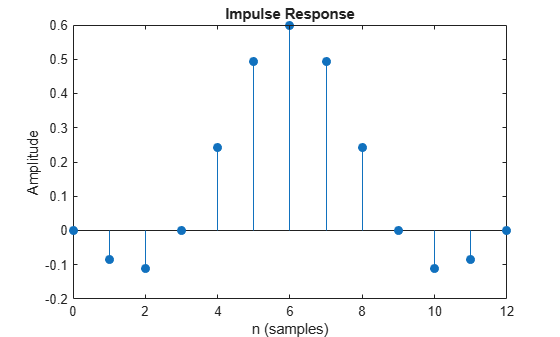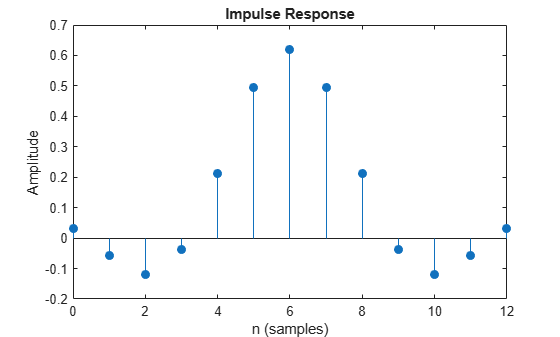rcosdesign

Raised cosine FIR pulse-shaping filter design

Description

example

b = rcosdesign(beta,span,sps) returns the coefficients, b, that correspond to a square-root raised cosine FIR filter with rolloff factor specified by beta. The filter is truncated to span symbols, and each symbol period contains sps samples. The order of the filter, sps*span, must be even. The filter energy is 1.

example

b = rcosdesign(beta,span,sps,shape) returns a square-root raised cosine filter when you set shape to 'sqrt' and a normal raised cosine FIR filter when you set shape to 'normal'.

Examples

collapse all

Specify a rolloff factor of 0.25. Truncate the filter to 6 symbols and represent each symbol with 4 samples. Verify that 'sqrt' is the default value of the shape parameter.

h = rcosdesign(0.25,6,4);
mx = max(abs(h-rcosdesign(0.25,6,4,'sqrt')))
mx = 0
fvtool(h,'Analysis','impulse')Compare a normal raised cosine filter with a square-root cosine filter. An ideal (infinite-length) normal raised cosine pulse-shaping filter is equivalent to two ideal square-root raised cosine filters in cascade. Thus, the impulse response of an FIR normal filter should resemble that of a square-root filter convolved with itself.

Create a normal raised cosine filter with rolloff 0.25. Specify that this filter span 4 symbols with 3 samples per symbol.

rf = 0.25;
span = 4;
sps = 3;

h1 = rcosdesign(rf,span,sps,'normal');
fvtool(h1,'impulse')The normal filter has zero crossings at integer multiples of sps. It thus satisfies Nyquist's criterion for zero intersymbol interference. The square-root filter, however, does not:

h2 = rcosdesign(rf,span,sps,'sqrt');
fvtool(h2,'impulse')Convolve the square-root filter with itself. Truncate the impulse response outward from the maximum so it has the same length as h1. Normalize the response using the maximum. Then, compare the convolved square-root filter to the normal filter.

h3 = conv(h2,h2);
p2 = ceil(length(h3)/2);
m2 = ceil(p2-length(h1)/2);
M2 = floor(p2+length(h1)/2);
ct = h3(m2:M2);

stem([h1/max(abs(h1));ct/max(abs(ct))]','filled')
xlabel('Samples')
ylabel('Normalized amplitude')
legend('h1','h2 * h2')The convolved response does not coincide with the normal filter because of its finite length. Increase span to obtain closer agreement between the responses and better compliance with the Nyquist criterion.

This example shows how to pass a signal through a square-root, raised cosine filter.

Specify the filter parameters.

rolloff = 0.25;     % Rolloff factor
span = 6;           % Filter span in symbols
sps = 4;            % Samples per symbol

Generate the square-root, raised cosine filter coefficients.

b = rcosdesign(rolloff, span, sps);

Create a vector of bipolar data.

d = 2*randi([0 1], 100, 1) - 1;

Upsample and filter the data for pulse shaping.

x = upfirdn(d, b, sps);

r = x + randn(size(x))*0.01;

Filter and downsample the received signal for matched filtering.

y = upfirdn(r, b, 1, sps);

For information on how to use square-root, raised cosine filters to interpolate and decimate signals, see Interpolate and Decimate Using RRC Filter (Communications Toolbox).

Input Arguments

collapse all

Rolloff factor, specified as a real nonnegative scalar not greater than 1. The rolloff factor determines the excess bandwidth of the filter. Zero rolloff corresponds to a brick-wall filter and unit rolloff to a pure raised cosine.

Data Types: double | single

Number of symbols, specified as a positive integer scalar.

Data Types: double | single

Number of samples per symbol (oversampling factor), specified as a positive integer scalar.

Data Types: double | single

Shape of the raised cosine window, specified as either 'normal' or 'sqrt'.

Output Arguments

collapse all

Raised cosine filter coefficients, returned as a row vector.

Data Types: double | single

Tips

• If you have a license for Communications Toolbox™ software, you can perform multirate raised cosine filtering with streaming behavior. To do so, use the System object™ filters, comm.RaisedCosineTransmitFilter and comm.RaisedCosineReceiveFilter.

 Tranter, William H., K. Sam Shanmugan, Theodore S. Rappaport, and Kurt L. Kosbar. Principles of Communication Systems Simulation with Wireless Applications. Upper Saddle River, NJ: Prentice Hall, 2004.